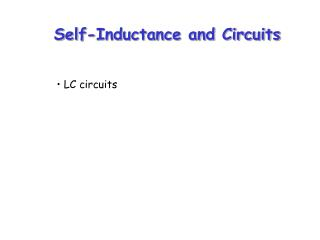Download PresentationSelf-Inductance and Circuits

# Self-Inductance and Circuits - PowerPoint PPT Presentation

Self-Inductance and Circuits. LC circuits. ε /R. 63%. I. t. 0 1 τ 2 τ 3 τ 4 τ. Recall : RC circuit, increasing current. Recall : RC circuit, decreasing current. I. I o. 0.37 I 0. t. 0 τ τ 2 τ 3 τ 4 τ. Question:.I am the owner, or an agent authorized to act on behalf of the owner, of the copyrighted work described.
Download Presentation## Self-Inductance and Circuits

Download Policy: Content on the Website is provided to you AS IS for your information and personal use and may not be sold / licensed / shared on other websites without getting consent from its author.While downloading, if for some reason you are not able to download a presentation, the publisher may have deleted the file from their server.

- - - - - - - - - - - - - - - - - - - - - - - - - - E N D - - - - - - - - - - - - - - - - - - - - - - - - - -
Presentation Transcript
1. Self-Inductance and Circuits • LC circuits

2. ε/R 63% I t 01τ 2τ 3τ 4τ Recall: RC circuit, increasing current

3. Recall: RC circuit, decreasing current I Io 0.37 I0 t 0τ τ 2τ 3τ 4τ

4. Question: What happens if we put a capacitor in the circuit, along with R, or L, or both?

5. LC circuits I • A capacitor is connected to an inductor in an LC circuit • Assume the capacitor is initially charged and then the switch is closed • Assume no resistance and no energy losses to radiation + C - L The switch is closed at t =0; Find I (t).

6. Oscillations in an LC Circuit, 1 • The capacitor is fully charged • The energy U in the circuit is stored in the electric field of the capacitor • The energy is equal to Q 2max/2C • The current in the circuit is zero • No energy is stored in the inductor • The switch is closed

7. Oscillations in an LC Circuit, 2 • The current is equal to the rate at which the charge changes on the capacitor (dQ/dt) • As the capacitor discharges, the energy stored in the electric field decreases • Since there is now a current, some energy is stored in the magnetic field of the inductor • Energy is transferred from the electric field to the magnetic field

8. Oscillations in an LC Circuit, 3 • The capacitor becomes fully discharged • It stores no energy • All of the energy is now stored in the magnetic fieldof the inductor • This energy is equal to ½ LI2 • The current reaches its maximum value • The current now decreases in magnitude, recharging the capacitor with its plates having opposite their initial polarity

9. Oscillations in an LC Circuit, 4 • Eventually the capacitor becomes fully charged and the cycle repeats • The energy continues to oscillate between the inductor and the capacitor • The total energy stored in the LC circuit remains constant in time and equals:

10. LC Circuit Analogy to Spring-Mass System • The kinetic energy (½mv2) of the spring is analogous to the magnetic energy (½L I 2) stored in the inductor • The potential energy ½kx2 stored in the spring is analogous to the electric potential energy ½CVmax2 stored in the capacitor • At any point in the cycle, energy is shared between the electric and magnetic fields

11. LC circuits The switch is closed at t =0; Find I (t). I + C - L Looking at the energy loss in each component of the circuit gives us:EL+EC=0 Which can be written as (remember, P=VI):

12. Solution

13. Charge and Current in an LC Circuit • The charge on the capacitor oscillates between Qmax and –Qmax • The current in the inductor oscillates between Imax and –Imax • Q and I are 90o out of phase with each other • So when Q is a maximum, I is zero, etc.

14. Energy in an LC Circuit – Graphs • The energy continually oscillates between the energy stored in the electric and magnetic fields • When the total energy is stored in one field, the energy stored in the other field is zero

15. Example 1: A 1.00-μF capacitor is charged by a 40.0-V power supply. The fully charged capacitor is then discharged through a 10.0-mH inductor. Find the maximum current in the resulting oscillations.

16. Example 2: An LC circuit consists of a 3.30-H inductor and an 840-pF capacitor, initially carrying a 105-μC charge. The switch is open for t < 0 and then closed at t = 0. Compute the following quantities at t = 2.00 ms: a) the energy stored in the capacitorb) the energy stored in the inductorc) the total energy in the circuit.

17. Solution

18. Example 3: The switch in the figure below is connected to point a for a long time. After the switch is thrown to point b, what are a) the frequency of oscillation of the LC circuit, b) the maximum charge that appears on the capacitor, c) the maximum current in the inductord) the total energy the circuit possesses at t = 3.00 s?

19. Solution

20. Notes About Real LC Circuits • In actual circuits, there is always some resistance • Therefore, there is some energy transformed to internal energy • The total energy in the circuit continuously decreases as a result of these processes